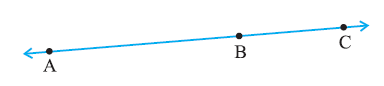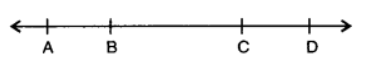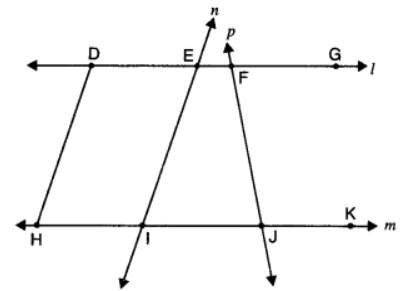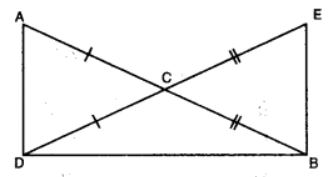Daily Practice Problems
Class 9 Maths
Introduction to Euclid’s GeometryQuestion 1:

Solve the equation a – 15 = 25 and state which axiom do you use here?

Question 2:

Ram and Ravi have the same weight. If they each gain weight by 2 kg, how will their new weights be compared?

Question 3:If A, B and C are three points on a line, and B lies between A and C as shown in

figure, then prove that AB + BC = AC.

Question 4:

If a point C be the mid-point of a line segment AB, then write the relation among AC, BC and AB.

Question 5:

How many lines do pass through two distinct points?

Question 6:

In the given figure, if AB = CD, then prove that AC = BD. Also, write the Euclid’s axiom used for proving it.Question 7:

Define: (a) Square                    (b) Perpendicular linesQuestion 8:

In the given figure, name the following:

(i) Four collinear points

(ii) Five rays

(iii) Five line segments

(iv) Two-pairs of non-intersecting line segments.

Question 9:

In the given figure, AC = DC and CB = CE. Show that AB = DE. Write the Euclid’s axiom to support this.Question 10:

Define each of the following terms

i. Angle                             ii. Line segment                         iii. Radius of a circle

Question 11:

What is Euclid’s fifth postulate?

Question 12:

Mohan and Sohan have the same weight. If they each gain weight by 2 kg, how will their new weights be compared?

Question 13:

The Euclidean geometry is valid only for figures in the _______.

Question 14:

Euclid’s fourth axiom says that _______.

Question 15:

Axioms are assumed

(a) Theorems

(b) Definitions

(c) Universal truths specific to geometry

(d) Universal truths in all branches of mathematics

Question 16:

Which of the following needs a proof?

(a) Definition

(b) Postulate

(c) Theorem

(d) Axiom

Question 17:

It is known that if x + y = 10 then x + y + z = 10 + z. Euclid’s axiom that illustrates this statement is

(a) First Axiom

(b) Second Axiom

(c) Third axiom

(d) Fourth Axiom

Question 18:

“Lines are parallel if they do not intersect” is stated in the form of

(a) Definition

(b) Proof

(c) Postulate

(d) Axiom

Question 19:

The things which are double of the same thing are

(a) equal

(b) unequal

(c) double of the same thing

(d) halves of the same thing

Question 20:

Which of the following statements are true?

(a) Only one line can pass through a single point.

(b) There is an infinite number of lines which pass through two distinct points.

(c) A terminated line can be produced indefinitely on both the sides

(d) If two circles are equal, then their radii are unequal.

**********